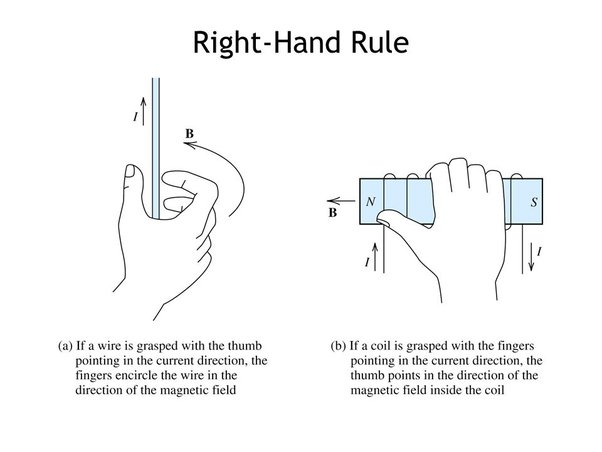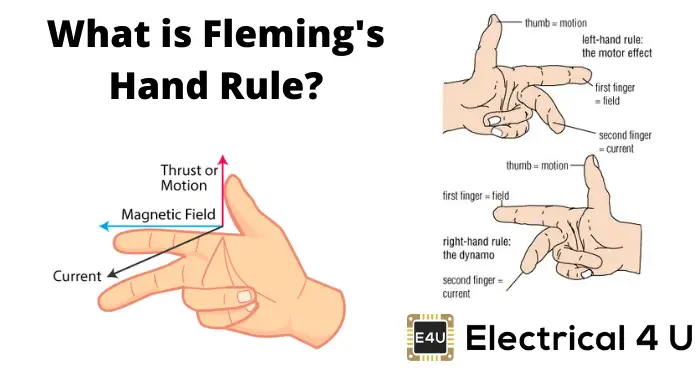# Fleming's Left Hand Rule

• Astrophysics• Electricity• Electromagnetism• Energy• Fields• Force• Mechanics• Momentum• Nuclear Physics• Quantities & Units• States of Matter• Waves• Key ExperimentsDid you know that when a wire with electricity flows through it is placed in a magnetic field, it experiences a force? This force is known as electromotive force or emf. This is the foundation of modern electric motors and generators. So, how do we know which way the force is going? Luckily, there is a simple method that can be used in almost all conditions. It's called Fleming's left-hand rule, discovered by John Ambrose Fleming in the late 19th century. This rule helps to find the direction of force, current, and magnetic field, and the best part is that all you need is your left hand!

## Definition of Fleming's Left-Hand Rule

Have you heard of Fleming's left-hand rule? This rule is easy to remember and can help you find the direction of force, current, and magnetic field. All you need is your left hand! Simply stretch out your thumb, middle finger, and index finger so that they make an angle. Then, your thumb will point to the force, your middle finger to the current, and your index finger to the magnetic field. You can even remember this with the acronym FBI, which stands for Force, current, and magnetic field. Let's try some example problems to see how it works.

## Examples of Fleming's left-hand rule

Imagine a wire across the computer screen, where the current flows from the left to the right of the screen and the external magnetic field is flowing from the top to the bottom of the screen. What will the direction of the force on the conductor be?Given the direction of the current and magnetic field, the force or thrust will point towards the screen

Using Fleming's left-hand rule, position the index finger and the middle finger in the direction of the magnetic field and the current, respectively. The thumb will be pointing towards the screen, so the force is directed into the screen. Imagine a wire placed on the ground, carrying a current that is flowing from south to north. It experiences a force to the east. Does the magnetic field point towards the ground or the sky?The direction of the external magnetic field can be found using Fleming's left-hand rule. by aligning the thumb and index finger in the direction of the current and force

Fleming's left-hand rule can be very useful in the context of electric motors. In an electric motor, a current-carrying wire is located inside a magnetic field. When the current flows through the wire, it experiences a force that causes it to move. The direction of this force can be determined using Fleming's left-hand rule. By placing the middle finger in the direction of the current, the index finger in the direction of the magnetic field, and the thumb in the direction of the force, you can determine the direction in which the wire will move. This is important for understanding how electric motors work and for designing them to function optimally.

Applying Fleming's left-hand rule

The forces acting on the current-carrying wire inside the electric motor, as determined using Fleming's left-hand rule, create a couple that causes the wire to rotate. This rotation is what drives the motor and allows it to perform its intended function. By applying the principles of electromagnetic induction and the laws of motion, electric motors are able to convert electrical energy into mechanical energy, making them essential components in many household appliances, vehicles, and industrial equipment.

## ‍

Fleming also has a right-hand rule, which is used in the context of electromagnetic induction.

Electromagnetic induction is the phenomenon due to which a current is induced in a conductor when the magnetic field around it fluctuates.

In the right-hand rule, the thumb indicates the direction of motion of the conductor, while the index finger indicates the direction of the magnetic field and the middle finger indicates the direction of the current. It's important not to confuse the thumb's function in the right-hand rule with its function in Fleming's left-hand rule, where it indicates the direction of the force on the conductor.

However, both the right-hand rule and Fleming's left-hand rule can be used to determine the relationship between the direction of the current, magnetic field, and resulting force or motion. By comparing the two hand rules, we can see that they are essentially the same, with the only difference being the function of the thumb.

## Importance of Fleming's left-hand rule

Fleming's left-hand rule is important because it provides a simple and accurate way of finding the direction of the force experienced by a current-carrying conductor in a magnetic field, given that you know the direction of both the current and the magnetic field. As we saw in the use case of an electric motor, using Fleming's left-hand rule, we can easily identify the direction in which the force acts on the arms of an electric motor. Considering that the workings of the all-important electromotor are well-understood in part due to Fleming's left-hand rule, we can conclude that Fleming's left-hand rule is, although indirectly, important in everyday life as well.

Fleming's Left-Hand Rule - Key takeawaysFleming's left-hand rule states that the thumb will point in the direction of the force, the index finger will point in the direction of the magnetic field, and the middle finger will point in the direction of the current. A mnemonic is FBI.Fleming's right-hand rule states that the thumb points in the direction of the motion of the conductor, the index finger points in the direction of the magnetic field, and the middle finger points in the direction of the induced current.Beware of the difference in the function of the thumb between the two hand rules of Fleming.Fleming's left-hand rule can be used to identify the direction of the forces in an electric motor.Fleming's right-hand rule can be used to identify the direction of the induced current in an electric generator.

## Fleming's Left Hand Rule

How to use Fleming's left-hand rule?

Fleming's left-hand rule is a rule that uses the left hand to find the direction of the force on a current-carrying conductor in a magnetic field. Stretch the thumb, index finger, and middle finger such that they're perpendicular to each other. The thumb points in the direction of the force (F), the middle finger in the direction of the current (I), and the index finger in the direction of the magnetic field (B).

What is Fleming's left-hand rule?

The rule states that if we stretch the thumb, middle finger, and index finger of the left hand in such a way that they make an angle of 90 degrees with each other, then the thumb will point in the direction of the force (F), the middle finger will point in the direction of the current (I), and the index finger will point in the direction of the magnetic field (B).

What is the difference between Fleming's left-hand and right-hand rule?

The right-hand rule is sometimes applied in the context of electromagnetic induction to find the direction of the induced current (I) through a conductor, whereas the left-hand rule is applied in the context of the motor effect to find the direction of the force (F) through a conductor. The right-hand rule is applied more in the case of electric generators, while the left-hand rule is applied in the case of electric motors.

What is the importance of Fleming's left-hand rule?

Fleming's left-hand rule is important because it provides an accurate way of finding the direction of the force experienced by a current-carrying conductor in a magnetic field.

What is an example of Fleming's left-hand rule?

An application of Fleming's left-hand rule is seen in the use case of an electromotor.14-day free trial. Cancel anytime.Join 10,000+ learners worldwide.The first 14 days are on us96% of learners report x2 faster learningFree hands-on onboarding & supportCancel Anytime